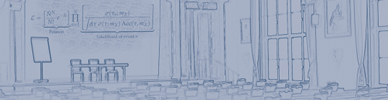#hadron2011

Jun 13 – 17, 2011
Künstlerhaus
Europe/Berlin timezone
Proceedings are now available online at <a href="http://www.slac.stanford.edu/econf/C110613/">eConf</a>

## Low-Energy Pion-Photon Reactions and Chiral Symmetry

Jun 13, 2011, 9:00 AM
30m
Festsaal (Künstlerhaus)

### Festsaal

#### Künstlerhaus

talk Plenary Session

### Speaker

Prof. Norbert Kaiser (TUM)

### Description

In this talk, I review the description of low-energy pion-Compton scattering in chiral perturbation theory. At one-loop order, the effects due to the pion structure consist of the electric and magnetic polarizabilities (subject to the constraint $\alpha_\pi+\beta_\pi =0$) and a unique pion-loop correction interpretable as photon scattering off the pion-cloud around the pion''. The latter compensates in the differential cross section $d\sigma/d\Omega_{cm}$ partly the reduction effects due to the polarizabilities \cite{picross}. The two-loop corrections to charged pion-Compton scattering, first calculated by Bürgi  and recently completed and reevaluated by Gasser et al.  are relatively small in the low-energy region $\sqrt{s}<4m_\pi$. Therefore chiral perturbation theory leads to firm predictions for the pion polarizabilities : $\alpha_\pi-\beta_\pi = (5.7\pm 1.0) \cdot 10^{-4} fm^3$ and $\alpha_\pi+ \beta_\pi = 0.16\cdot 10^{-4} fm^3$. Because of the smallness of the pion-structure effects (less than 20 %) radiative corrections of order $\alpha$ have to be included also in the analysis of pion-Compton scattering data. It is found that these QED radiative corrections \cite{radcor} have the same kinematical signature as the polarizability difference $\alpha_\pi-\beta_\pi$, but their effects are suppressed in magnitude by about a factor 5 or more. The other topic of my talk is the description of the (charged and neutral) pion-pair photoproduction processes $\pi^-\gamma \to \pi^- \pi^0\pi^0$ and $\pi^-\gamma \to \pi^+ \pi^-\pi^-$ at next-to-leading order in chiral perturbation theory . Whereas the total cross section $\sigma_{tot}(s)$ for the neutral channel $\pi^-\gamma \to \pi^- \pi^0\pi^0$ gets enhanced sizeably by the inclusion of chiral loop and counterterm corrections, the analogous effects turn out to be very small for the charged channel $\pi^-\gamma \to \pi^+ \pi^-\pi^-$. This different behavior can be understood from the varying influence of the chiral corrections on the pion-pion final state interaction ($\pi^+\pi^-\to \pi^0\pi^0$ versus $\pi^-\pi^- \to \pi^-\pi^-$). The QED radiative corrections have also been calculated for the process $\pi^-\gamma \to \pi^- \pi^0\pi^0$ (simpler case) . These affect the total cross section by at most 2 %. The predictions of chiral perturbation theory for low-energy pion-photon reactions can be tested soon by the COMPASS experiment at CERN which uses Primakoff scattering of high-energy pions in the Coulomb-field of a heavy nucleus. A preliminary analysis for $\pi^-\gamma \to \pi^+ \pi^-\pi^-$ in the energy region $3m_\pi < \sqrt{s} < 5m_\pi$ confirms the prediction of chiral perturbation theory.  U. Bürgi, Nucl. Phys. B479 (1996) 392; Phys. Lett. B377 (1996) 147.  J. Gasser, M.A. Ivanov and M.E. Sainio, Nucl. Phys. B745 (2006) 84.  N. Kaiser, Nucl. Phys. A848 (2010) 198; Eur. Phys. J. A46 (2010) 373.  N. Kaiser and J.M. Friedrich, Eur. Phys. J. A36 (2008) 181.  N. Kaiser and J.M. Friedrich, Nucl. Phys. A812 (2008) 186.

### Primary author

Prof. Norbert Kaiser (TUM)

 Slides